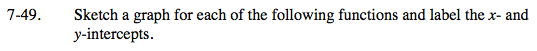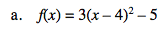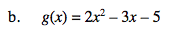Home > INT3 > Chapter 7 > Lesson 7.1.4 > Problem7-49

7-49.
1. Sketch a graph for each of the following functions and label the x‑ and y‑intercepts. Homework Help ✎

1. f(x) = 3(x – 4)2 – 5

2. g(x) = 2x2 – 3x – 5The equation of this quadratic function is in graphing form. Where is its vertex?Rewrite this equation in factored form. What are the x-intercepts? (You should be able to tell once the equation is in factored form.)